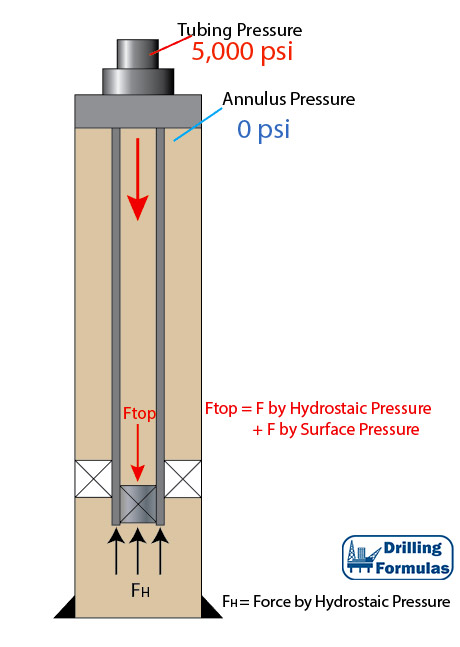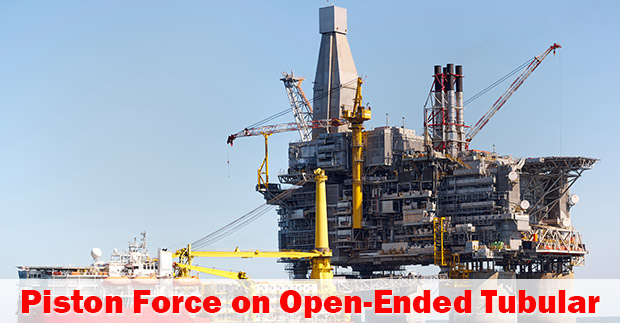## Piston Force on Closed-Ended Tubular (Plugged Tubular)According to the previous post (piston force on open-ended tubular), applied surface pressure will reduce tensile force on surface. In this article, this is an analysis on the piston force on a plugged tubular string and the details are shown below;

Tubing Detail

• 5” Tubing
• ID of tubing = 3.696”
• Packer seal bore OD = 5.25”
• Weight per length = 17.7 lb/ft
• Total Length = 10,000 ft
• Plugged tubing depth = 10,000 ft
• Fluid density = 10 ppg
• Tubing is free to move in the packer
• Applied surface pressure = 5,000 psi

Figure 1 shows the wellbore schematic. Applied pressure (5,000 psi) will cause a piston effect to push the tubing. Therefore, at the bottom of tubing buoyancy and piston force will act in an upwards direction (compression).Figure 1 – Wellbore Schematic

## Piston Force on Open-Ended TubularPiston force is a load caused directly by changes in pressure acting on the exposed cross sectional area of pipe. This results in changing in length of tubular and force acting against tubular. In this article, it generally demonstrates force distribution based on a simple tubular diagram.Tubing Detail

• 5” Tubing
• ID of tubing = 3.696”
• Weight per length = 17.7 lb/ft
• Total Length = 10,000 ft
• Fluid density = 10 ppg
• Tubing is free to move in the packer
• Applied surface pressure = 5,000 psi